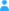Sign in

|

Dependencies in Dataset #3054

during ACRIDICON-CHUVA mission

Entries (observed variables)

Information about observed variables of the current dataset.
No. Name
01 elapsed UT seconds from 0 hours on day line 7
02 Altitude = GPS altitude (km)
03 Org = organic aerosol mass concentration (ug per STD cubic m)
04 Sigma_Org = uncertainty of Org (ug per STD cubic m)
05 DL_Org = detection limit of Org (ug per STD cubic m)
06 NO3 = nitrate aerosol mass concentration (ug per STD cubic m)
07 Sigma_NO3 = uncertainty of NO3 (ug per STD cubic m)
08 DL_NO3 = detection limit of NO3 (ug per STD cubic m)
09 SO4 = sulfate aerosol mass concentration (ug per STD cubic m)
10 Sigma_SO4 = uncertainty of SO4 (ug per STD cubic m)
11 DL_SO4 = detection limit of SO4 (ug per STD cubic m)
12 NH4 = ammonium aerosol mass concentration (ug per STD cubic m)
13 Sigma_NH4 = uncertainty of NH4 (ug per STD cubic m)
14 DL_NH4 = detection limit of NH4 (ug per STD cubic m)

Dependencies of the observed variables

The interactive table provides a visualization of the dependencies of this dataset's entries (variables) which will hopefully assist you to understand the datasets structure.

Most datasets are simple, e.g. like 10 entries depending on 1 entry. But there are other datasets which are far more complex; with relationships between various entries and even reversed dependencies.

Warning: It works only with JavaScript enabled in your browser.

Direction of relationships

Entry A (=depends on)

Explanation

Enable Javascript and you'll see the dependencies of this dataset explained in this box while hovering over the table on the left.

-->